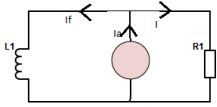Courses

# Test: Circuit Model of DC Machines

## 10 Questions MCQ Test Electrical Machines | Test: Circuit Model of DC Machines

Description
This mock test of Test: Circuit Model of DC Machines for Electrical Engineering (EE) helps you for every Electrical Engineering (EE) entrance exam. This contains 10 Multiple Choice Questions for Electrical Engineering (EE) Test: Circuit Model of DC Machines (mcq) to study with solutions a complete question bank. The solved questions answers in this Test: Circuit Model of DC Machines quiz give you a good mix of easy questions and tough questions. Electrical Engineering (EE) students definitely take this Test: Circuit Model of DC Machines exercise for a better result in the exam. You can find other Test: Circuit Model of DC Machines extra questions, long questions & short questions for Electrical Engineering (EE) on EduRev as well by searching above.
QUESTION: 1

Solution:

I = (V-E)/r
= (220-200)/1
=20 A.

QUESTION: 2

### The shaft power at the DC Generator is

Solution:

The shaft power is sum of mechanical power and rotational losses.

QUESTION: 3

### If the electromagnetic torque in a DC shunt-generator is opposite, what can be further concluded?

Solution:

When the electromagnetic torque is in opposite direction, it is of motoring nature.

QUESTION: 4

The conductor EMF and current are in _____ direction and developed torque is in _____ for generating mode.

Solution:

The conductor emf and current will be in same direction and the developed torque is in opposite direction for a generator.

QUESTION: 5

If the armature terminal voltage is more that its induced EMF, the DC machine given is

Solution:

As the terminal voltage is lesser than armature voltage, the supply is fed to the machine and so it will be acting like a motor.

QUESTION: 6

Consider a 200V, 25kW, 30A DC machine lap connected with armature resistance of 0.4 ohms. If the machine is later wave wound, then the developed power is

Solution:

The power of the machine remains unaltered by the type of connections.

QUESTION: 7

If the DC machine is held constant at 3000 rpm. The DC voltage is 250V. If the field is held constant with 250V. Is this machine generator or motor?

Solution:

From the speed and emf relation, E = 250*2950/3000
= 245.8 V
This is less than the terminal voltage. Hence it is a motor.

QUESTION: 8

A shunt generator has an induced voltage on open circuit of 127 V. When the machine is on load the terminal voltage is 120 V. The load current if the field resistance be 15 ohm and armature resistance be 15 ohm

Solution:

Ia*Ra = E-V
= 127 – 120
= 7 V
Ia = 350 A
Ish = 120/15
= 8 A
I = 350 – 8
= 342 AQUESTION: 9

The circuit depicting the equation V=Ea + I*Ra.

Solution:

This is a motor performance equation.

QUESTION: 10

The voltage drop at brush-commutator contact is variable (1-2V) and dependent of armature current.​

Solution:

The voltage drop at brush-commutator contact is variable (1-2V) and independent of armature current.